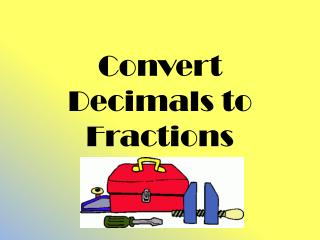DownloadDownload PresentationConvert Decimals to Fractions

Convert Decimals to Fractions

Télécharger la présentationConvert Decimals to Fractions

- - - - - - - - - - - - - - - - - - - - - - - - - - - E N D - - - - - - - - - - - - - - - - - - - - - - - - - - -
Presentation Transcript

1. Convert Decimals to Fractions

2. Place Value Review Tens Ones . Tenths Hundredths Thousandths Ten Thousandths 15 . 7456

3. What I already know… 0.5 = ½ 0.75 = ¾0.125 = 1/8 Use the place value of the last digit to determine the denominator. Drop the decimal and use that number as the numerator. • In the decimal 0.5 the “5” is in the tenths place so the denominator will be “10.” • The numerator will be 5. So the fraction is 5/10 which reduces to ½. • In the decimal 0.75 the last digit is in the hundredths place so the denominator will be “100.” • The numerator will be 75. So the fraction is 75/100 which reduces to ¾. • In the decimal 0.125 the last digit is in the thousandths place so the denominator will be “1000.” • The numerator will be 125. So the fraction is 125/1000 which reduces to 1/8.